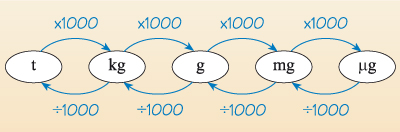Science, Maths & Technology

### Become an OU studentDiscovering chemistry

Start this free course now. Just create an account and sign in. Enrol and complete the course for a free statement of participation or digital badge if available.

# 1.2 Units of mass

You will have noticed that the term mass in the title of this section. Mass is a measure of how much matter an object contains, and is the correct term to use rather than weight. However the terms weigh and weight are used in general day to day parlance.

Weight [Tip: hold Ctrl and click a link to open it in a new tab. (Hide tip)] is actually the gravitational force experienced by an object (measured in newtons):

Weight = mass × acceleration due to gravity

Mass is measured in kilograms (kg), which again is not the most appropriate unit for laboratory use; chemists tend to weigh in grams (g, one thousandth of a kilogram) or milligrams (mg, one thousandth of a gram).

• A litre of water weighs 1 kg.
• A £20 note weighs about 1 g.
• A UK pound coin weighs around 10 g.

Originally a ‘pound’ was the monetary value given to a pound mass of sterling silver (an alloy of 92.5% silver and 7.5% copper), hence ‘pound sterling’.

• Express 120 mg in grams

• 120 mg = 0.120 g

In practice, the most commonly used units differ from each other by a factor of 1000. For example, in Figure 1 you can see how the units of mass – tonne (t), kilogram (kg), gram (g), milligram (mg) and microgram (µg) – differ from each other by a factor of 1000.Figure 1 Common units of measurement of weight